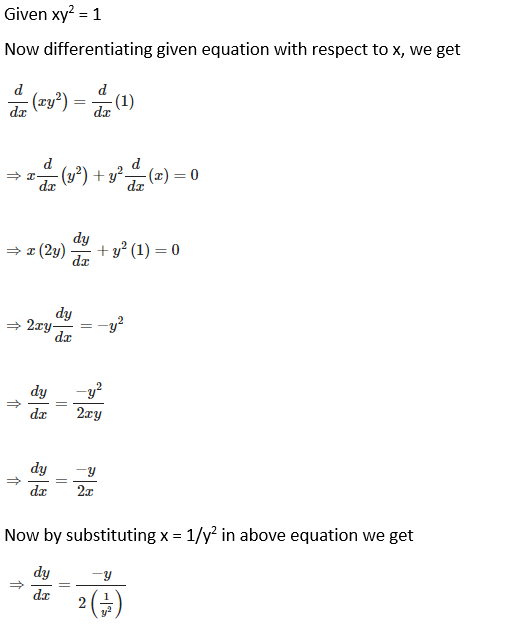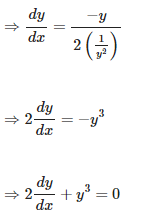# RD Sharma Solutions For Class 12 Maths Exercise 11.4 Chapter 11 Differentiation

RD Sharma Solutions Class 12 Maths Exercise 11.4 Chapter 11 Differentiation is provided here. In calculus, a method called implicit differentiation makes use of the chain rule to differentiate implicitly defined functions. By practising this exercise, students will learn how to differentiate the given implicit function by using the chain rule.

Students can refer to the PDF of RD Sharma Solutions as a major study material to improve their speed in solving problems accurately. Students can access RD Sharma Solutions Class 12 Maths Chapter 11 Differentiation Exercise 11.4 free PDF, which is given here. These solutions are prepared by expert faculty of BYJU’S to provide the best study material for students who aspire to score well in the upcoming board exams.

## RD Sharma Solutions For Class 12 Chapter 11 Differentiation Exercise 11.4:-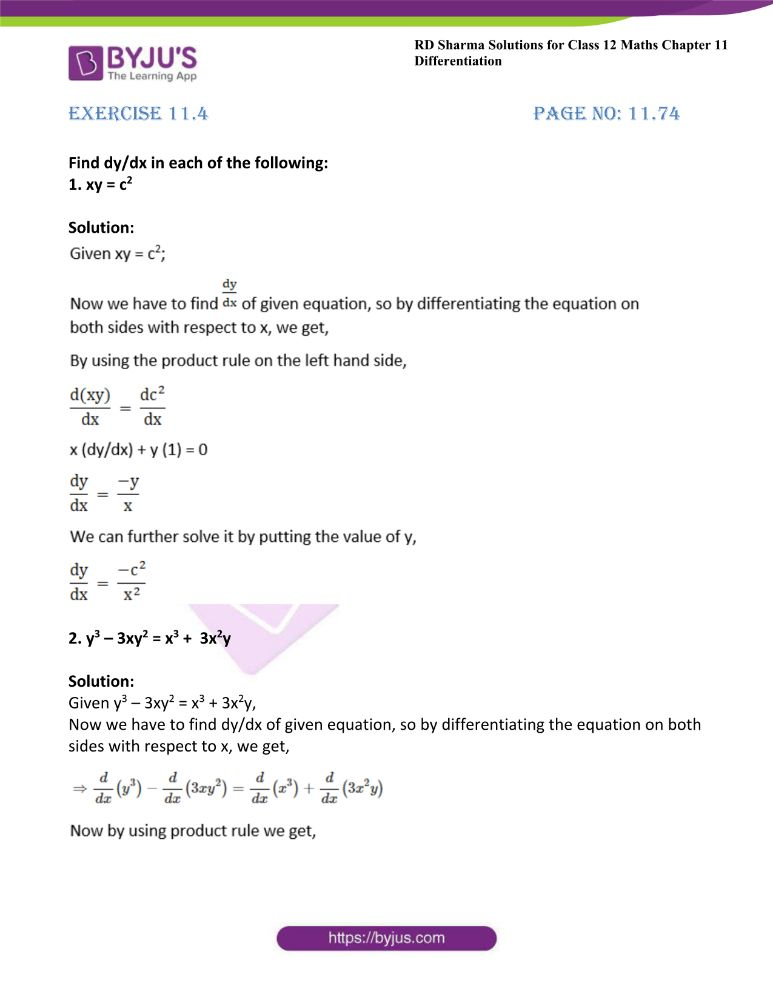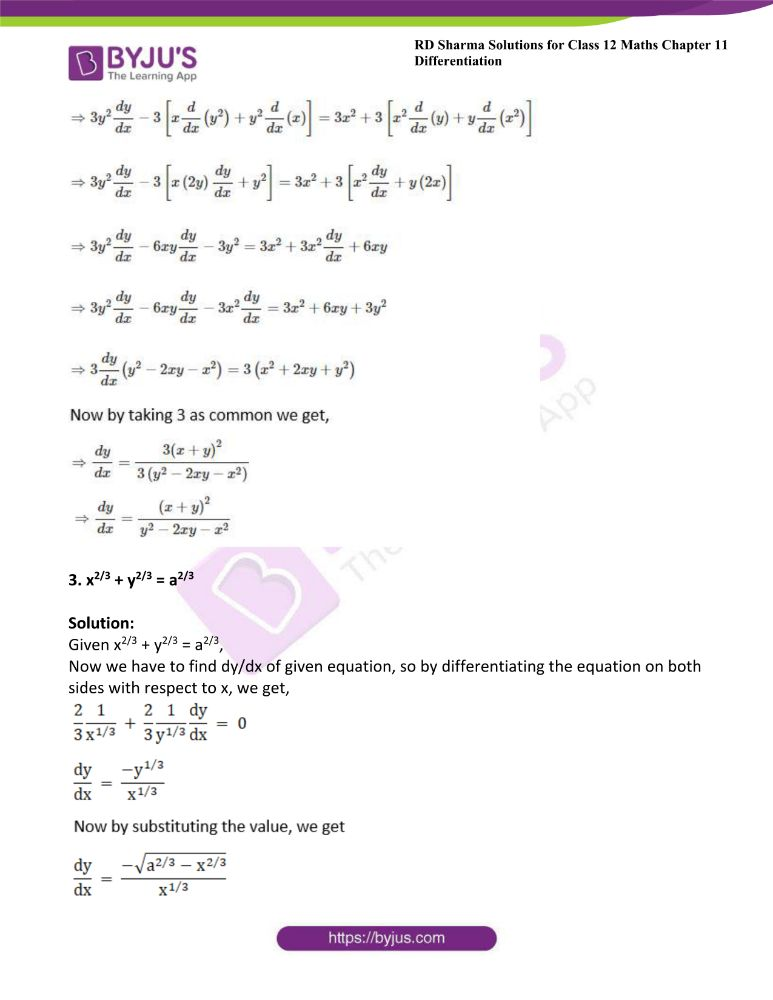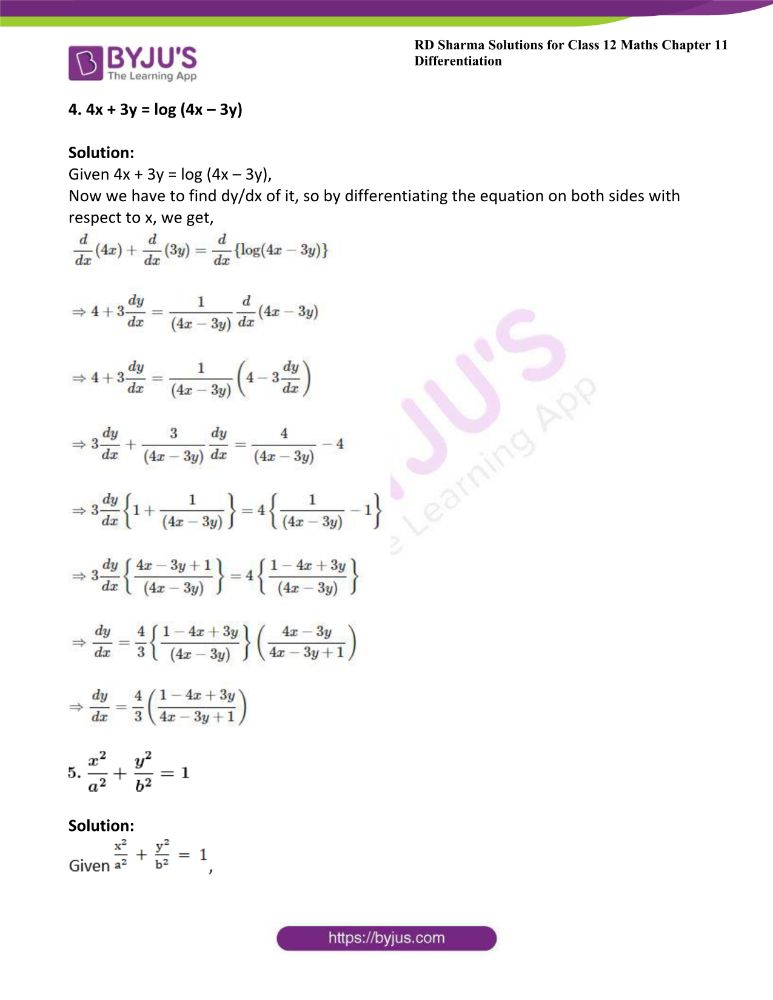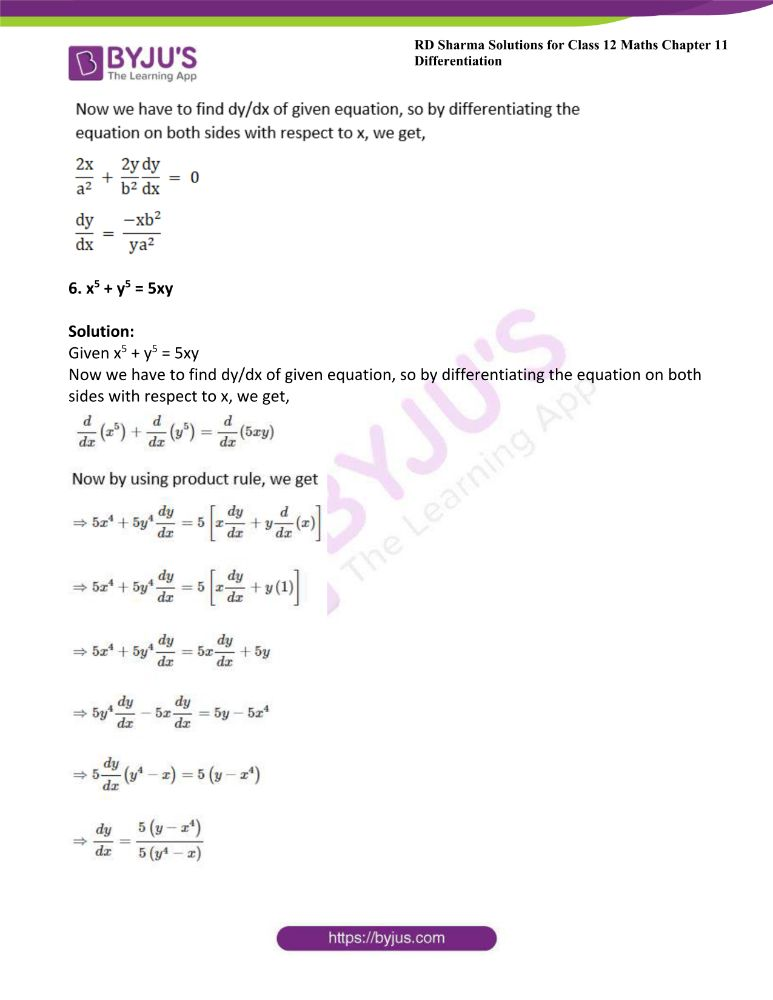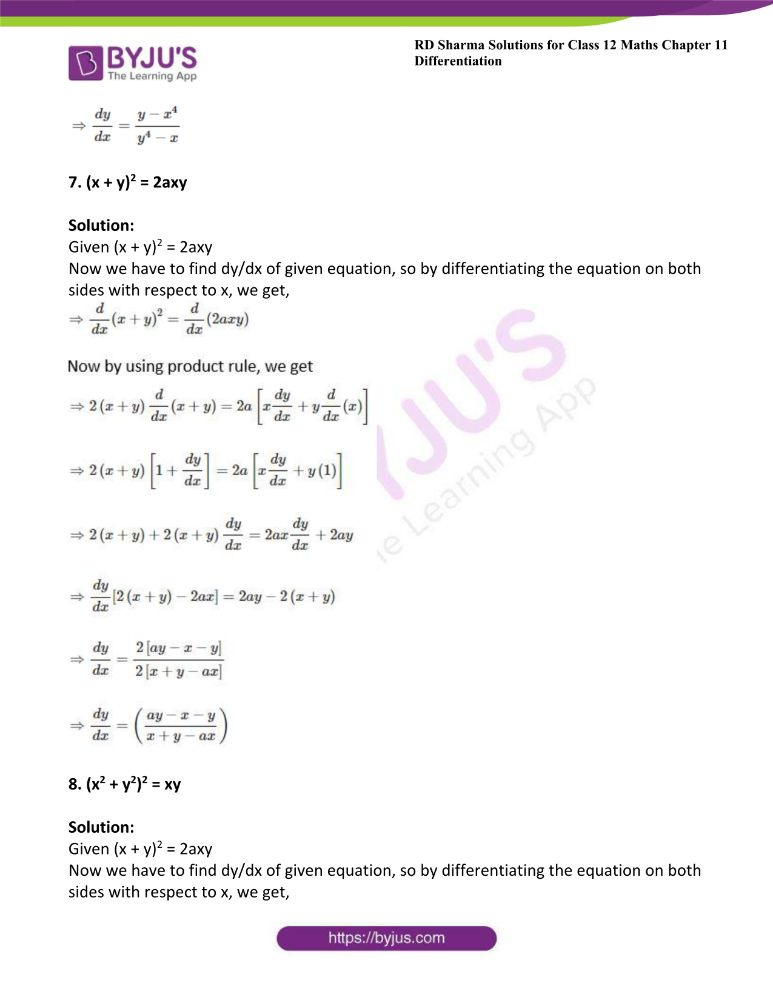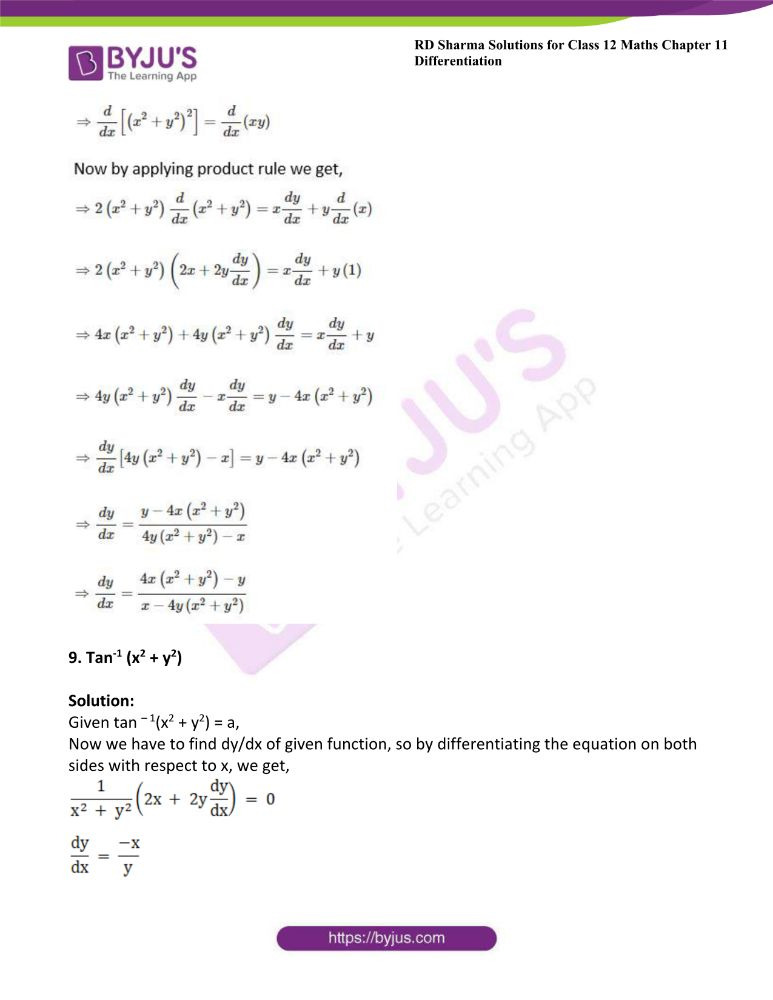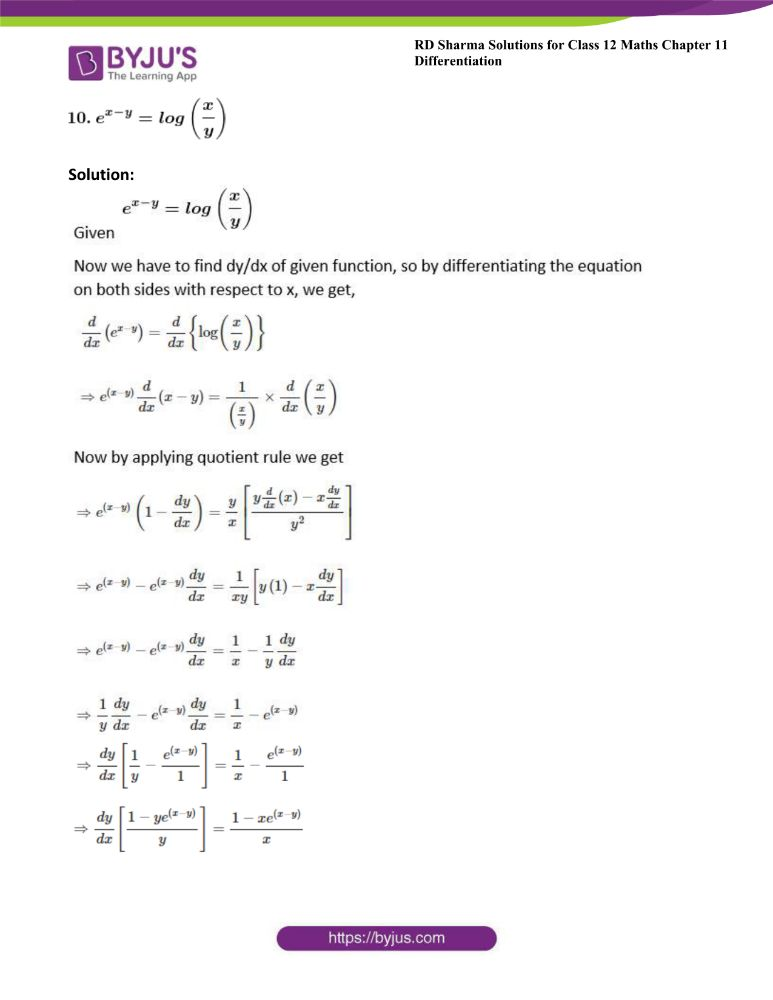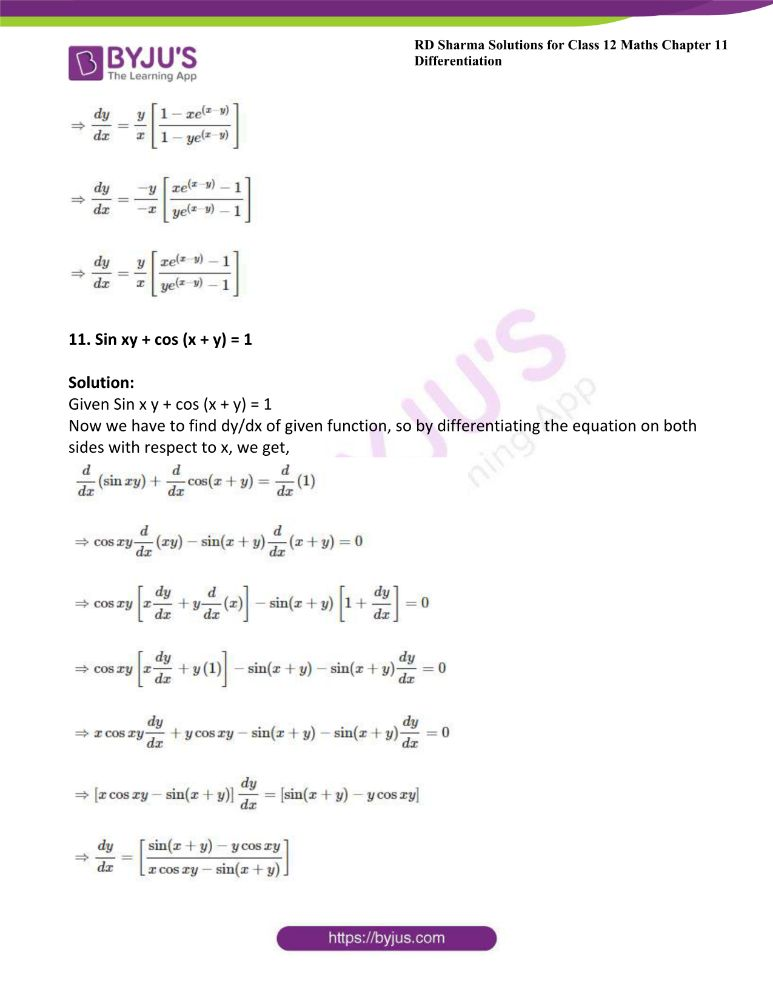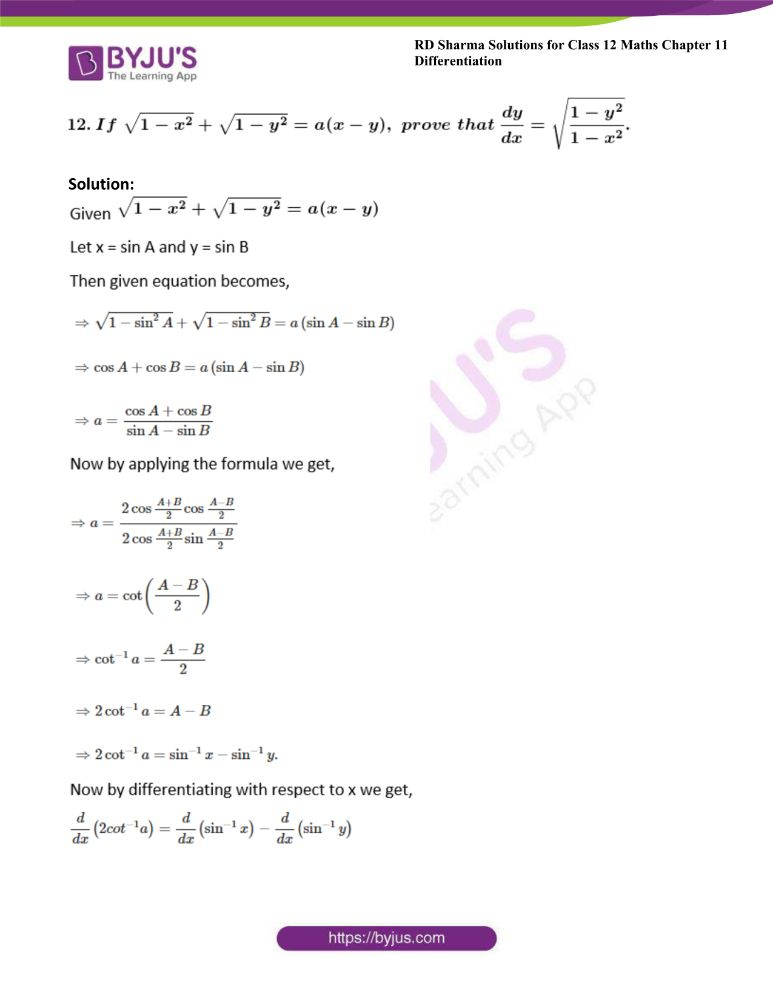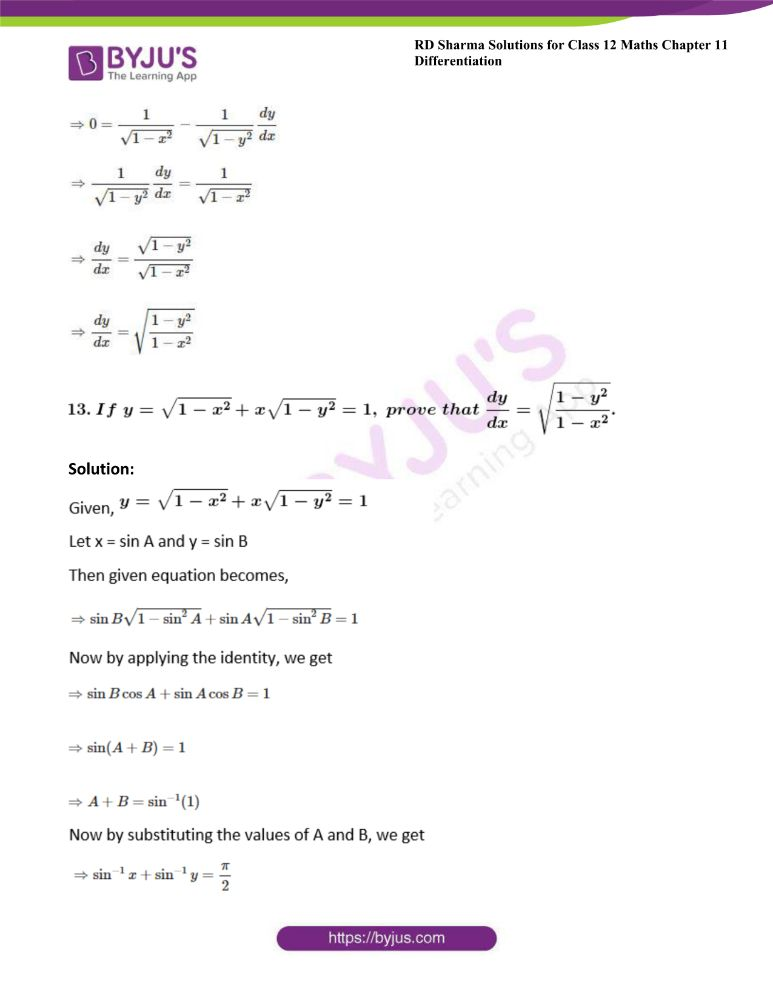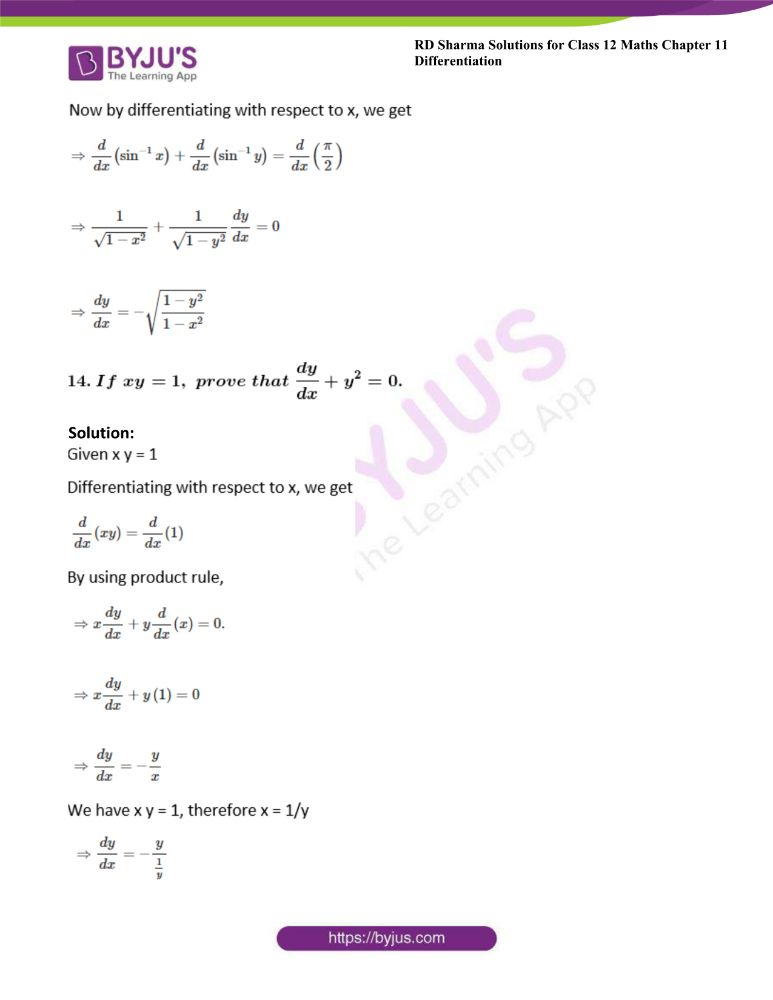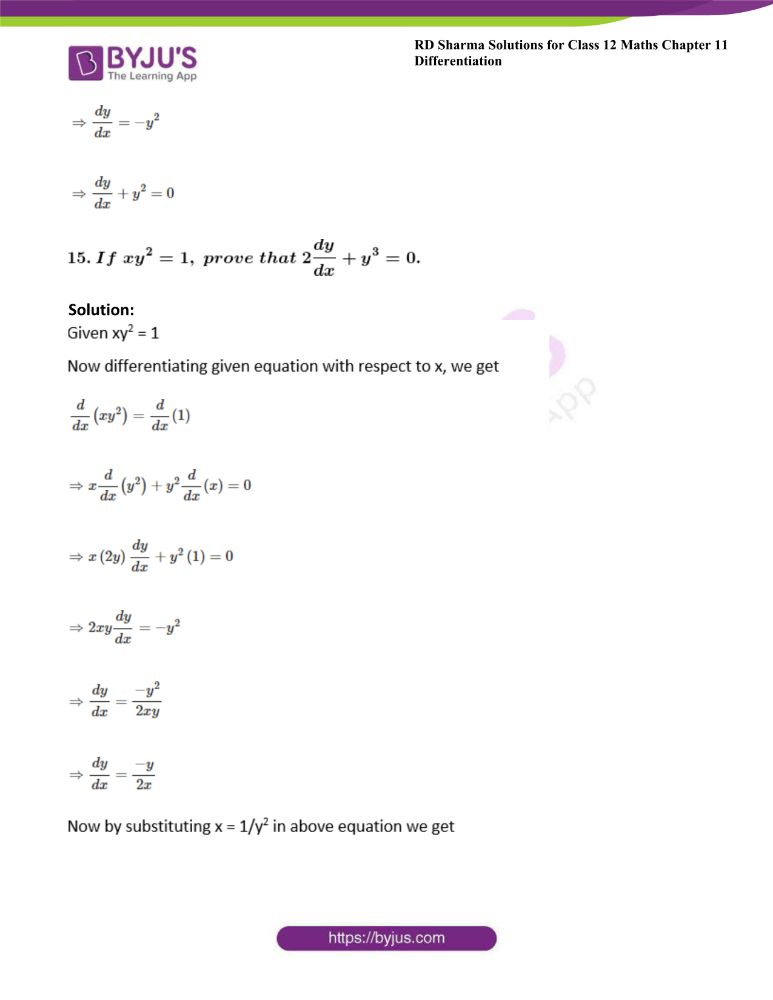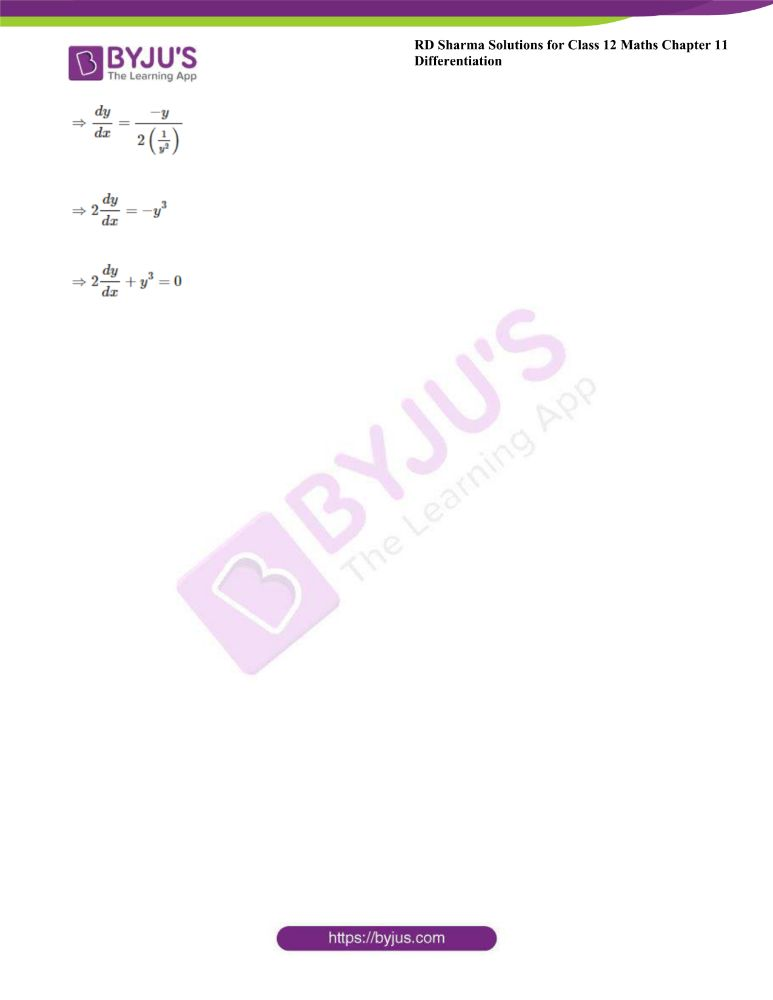### Access other exercises of RD Sharma Solutions For Class 12 Chapter 11 – Differentiation

Exercise 11.1 Solutions

Exercise 11.2 Solutions

Exercise 11.3 Solutions

Exercise 11.5 Solutions

Exercise 11.6 Solutions

Exercise 11.7 Solutions

Exercise 11.8 Solutions

### Access answers to Maths RD Sharma Solutions For Class 12 Chapter 11 – Differentiation Exercise 11.4

Exercise 11.4 Page No: 11.74

Find dy/dx in each of the following:

1. xy = c2

Solution: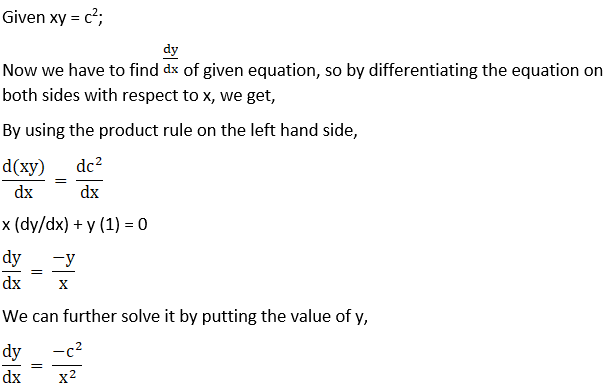2. y3 – 3xy2 = x3 + 3x2y

Solution:

Given y3 – 3xy2 = x3 + 3x2y,

Now we have to find dy/dx of given equation, so by differentiating the equation on both sides with respect to x, we get,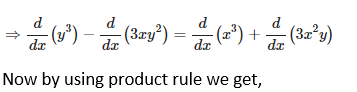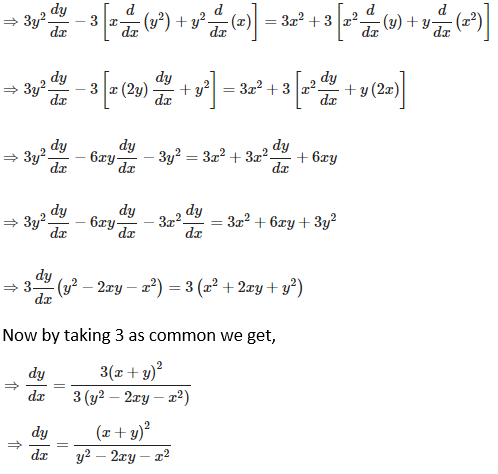3. x2/3 + y2/3 = a2/3

Solution:

Given x2/3 + y2/3 = a2/3,

Now we have to find dy/dx of given equation, so by differentiating the equation on both sides with respect to x, we get,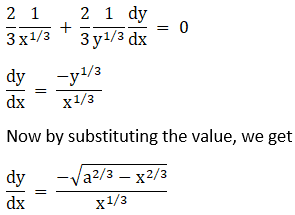4. 4x + 3y = log (4x – 3y)

Solution:

Given 4x + 3y = log (4x – 3y),

Now we have to find dy/dx of it, so by differentiating the equation on both sides with respect to x, we get,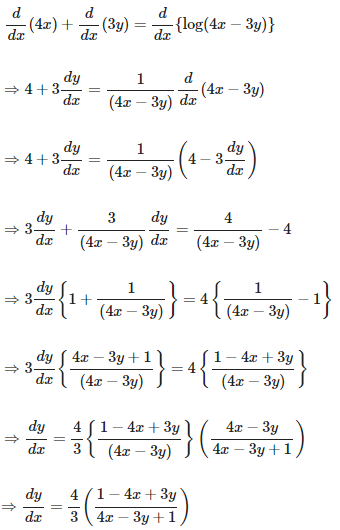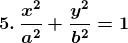Solution: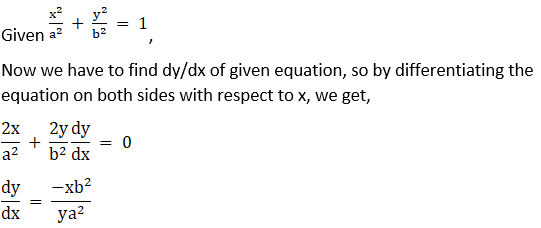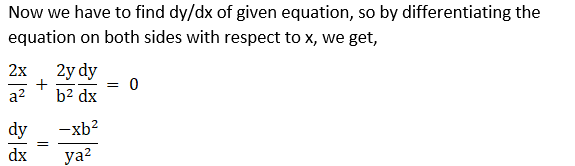6. x5 + y5 = 5xy

Solution:

Given x5 + y5 = 5xy

Now we have to find dy/dx of given equation, so by differentiating the equation on both sides with respect to x, we get,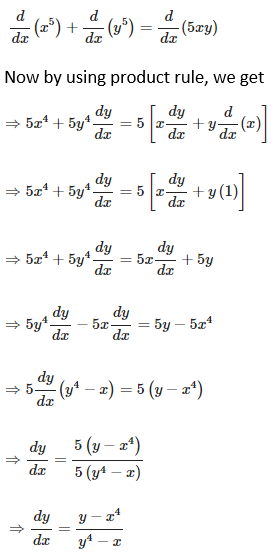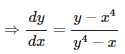7. (x + y)2 = 2axy

Solution:

Given (x + y)2 = 2axy

Now we have to find dy/dx of given equation, so by differentiating the equation on both sides with respect to x, we get,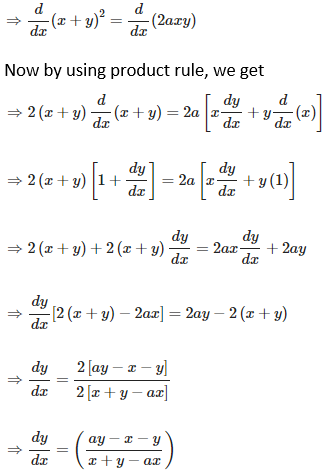8. (x2 + y2)2 = xy

Solution:

Given (x + y)2 = 2axy

Now we have to find dy/dx of given equation, so by differentiating the equation on both sides with respect to x, we get,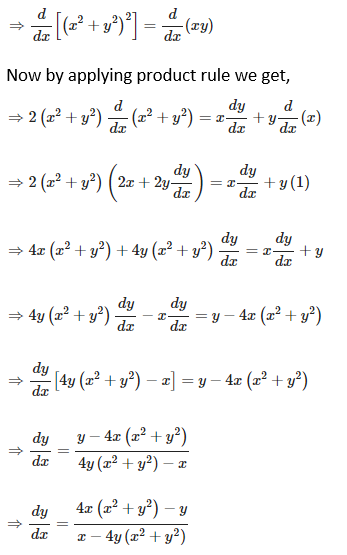9. Tan-1 (x2 + y2)

Solution:

Given tan – 1(x2 + y2) = a,

Now we have to find dy/dx of given function, so by differentiating the equation on both sides with respect to x, we get,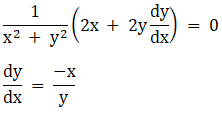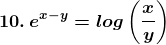Solution: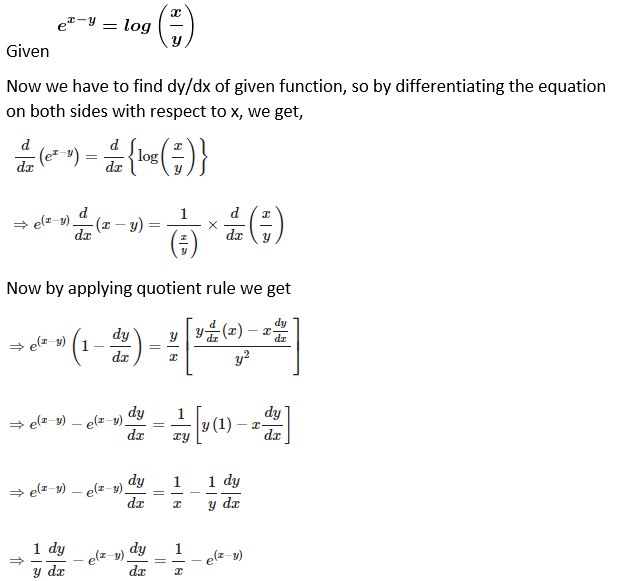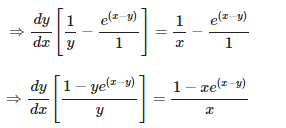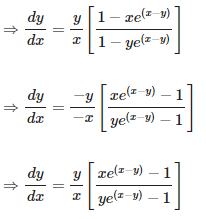11. Sin xy + cos (x + y) = 1

Solution:

Given Sin x y + cos (x + y) = 1

Now we have to find dy/dx of given function, so by differentiating the equation on both sides with respect to x, we get,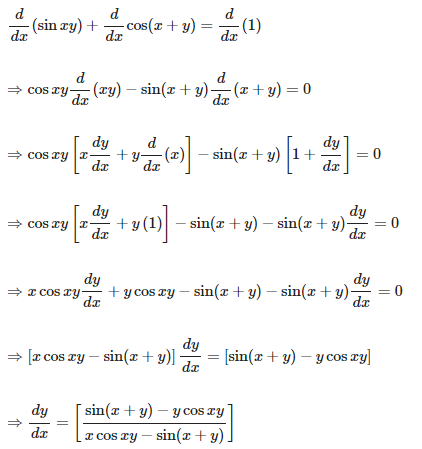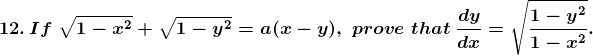Solution: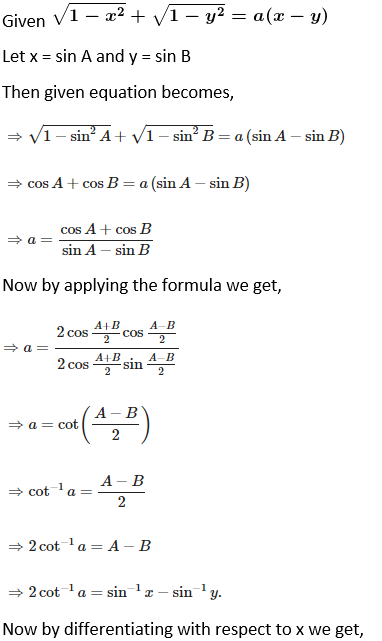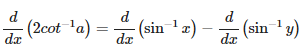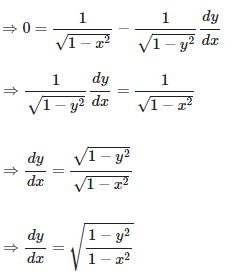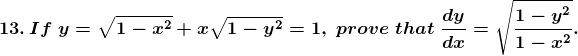Solution: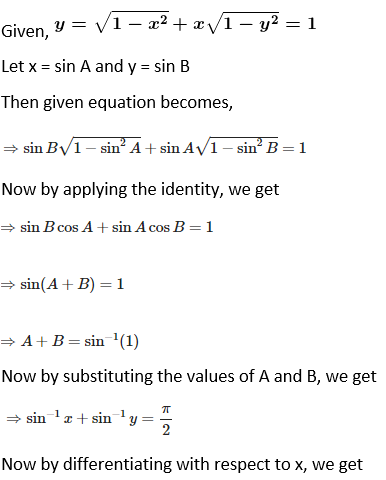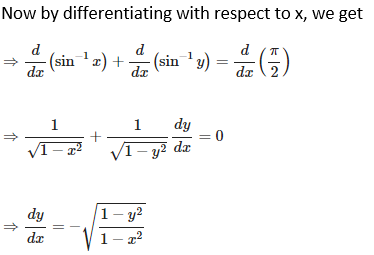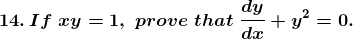Solution: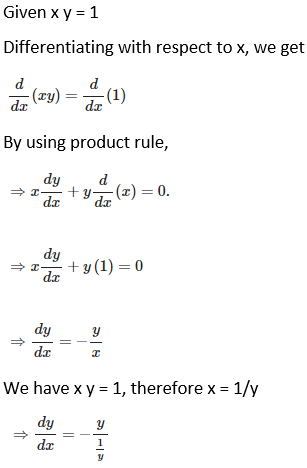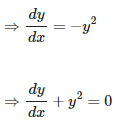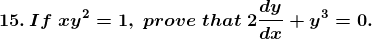Solution: